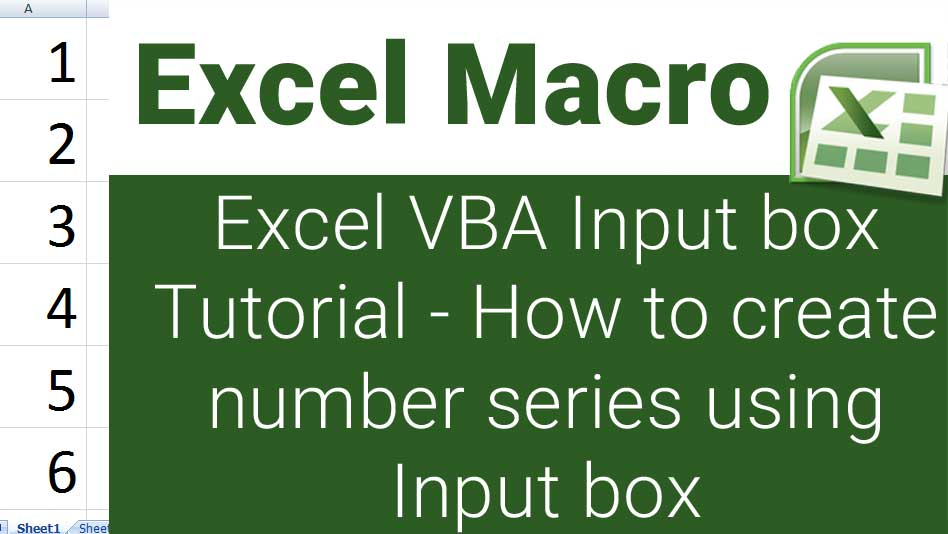# Excel VBA Input box tutorial – How to create number series using input box

Hello everyone this is an Excel VBA Input box tutorial on How to create number series using input box. Please go through the below detailed video tutorial for a better step by step instructions. This tutorial also offers the best example on For Next loop in excel VBA. The very important thing is that you can create number series with row and column gaps that is you could create 1 or more empty rows between the series. You cannot do this stuff with the default series dialog nor you can achieve this by dragging the series. Hence this is an awesome macro code that surely you will like. You can also use this for your macro project work. If you like this work please show me your support by subscribing my YouTube channel.

## Copy the code below for creating number series down the rows using inputbox function:

Sub SeriesInRows()
Dim X, Y, A, B, N
X = InputBox(“Enter the Row Offset”, “(Optional) Empty cells between series”, 0)
A = InputBox(“Enter the starting number in the series”, “Starting Number”, 1)
B = InputBox(“Enter the ending number in the series”, “Ending Number”, 50)
For N = A To B
ActiveCell.Value = N
ActiveCell.Offset(X + 1, Y).Select
Next N
X = 0
Y = 0
End Sub

## Copy the code below for creating number series across the columns using inputbox function:

Sub SeriesInColumns()
Dim X, Y, A, B, N
Y = InputBox(“Enter the Row Offset”, “(Optional) Empty cells between series”, 0)
A = InputBox(“Enter the starting number in the series”, “Starting Number”, 1)
B = InputBox(“Enter the ending number in the series”, “Ending Number”, 50)
For N = A To B
ActiveCell.Value = N
ActiveCell.Offset(X, Y + 1).Select
Next N
X = 0
Y = 0
End Sub

I am very sure that excel vba input box tutorial could be a best example for excel vba input box examples.### himaghiri

Himaghiri Thanayan.N is the founder of HBN Infotech. He has great dreams and passion for Graphic Design, Web Design, CAD Design, Arts & Crafts, Science & Information Technology and Article Writing.

1.Mandar says:

I can’t understand the first line.
Dim X, Y, A, B, N
I am a beginner in EXCEL VBA. I haven’t come accross such a Dim Statement. I am familiar with DIM X as Integer, Y as Range, etc.
Is all these are variants? Why not defined as integer?

2.M Lawrance says:

I want vba code for the following scenario

I want a input a box where user will be asked has to choose the range of cells.
then systme shall apply the cell borders(For e.g. “xldot”) to these selected cells

can you help me to get the query

This site uses Akismet to reduce spam. Learn how your comment data is processed.